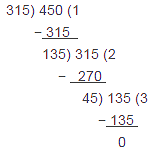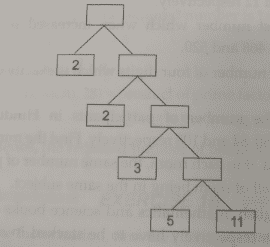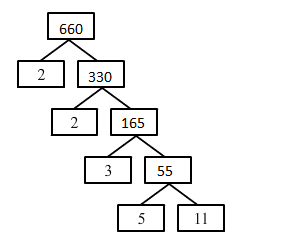RS Aggarwal Class 10 Solutions Chapter 1- Real Numbers Ex 1A

RS Aggarwal Class 10 Ex 1A

The RS Aggarwal Class 10 Maths textbook has a collection of important questions for practice. These solutions are helpful for students from the exam point of view. While solving the RS Aggarwal Class 10 Maths exercise question students can refer to these solutions if they get stuck while solving any problem. It will help you in scoring good marks in your exam. All the solutions provided here are in pdf format so that students can download it for future reference.

Practicing these solutions will make you feel more confident while attempting the final CBSE Class 10 board exam. It covers the entire Maths syllabus of Class 10 and for each topic, different types of questions are framed for students to practice. As the RS Aggarwal Maths textbook is designed as per the CBSE guidelines there is a chance that some questions might be picked up from the reference textbook.

Download PDF of RS Aggarwal Class 10 Solutions Chapter 1 – Real Number Ex 1A

Q.1: The HCF of two numbers is 145 and their LCM is 2175. If one of the numbers is 725, find the other.

Sol:

HCF of two numbers = 145

LCM of two numbers = 2175

Let one of the two numbers be 725 and other be x.

Using the formula, Product of two numbers = HCF x LCM

We conclude that:

725. X = 145 x 2175

X= $\frac{145X2175}{725}$ = 435

Hence, the other number is 435.

Q.2: Find the simplest form of:

(i) $\frac{ 69 }{ 92 }$

(ii) $\frac{ 473 }{ 645 }$

(iii) $\frac{ 1095 }{ 1168 }$

(iv) $\frac{ 368 }{ 496 }$

Sol:

(i) Prime factorization of 69 and 92 is:

69 = 3 x 23

92 = 22 x 23

Therefore, $\frac{69}{92}$= $\frac{3X23}{2^{2}X23}$ = $\frac{3}{2^{2}}$= $\frac{3}{4}$

Thus, simplest form of $\frac{69}{92}$ is $\frac{3}{4}$.

(ii) Prime factorization of 473 and 645 is:

473 = 11 x 43

645 = 3 x 5 x 43

Therefore, $\frac{473}{645}$ =$\frac{11X43}{3X5X43}$= $\frac{11}{15}$

Thus, simplest form of $\frac{473}{645}$ is $\frac{11}{15}$.

(iii) Prime factorization of 1095 and 1168 is:

1095=3 x 5 x 73

1168=24x73

Therefore, $\frac{1095}{1168}$= $\frac{15}{16}$

Thus, simplest form of $\frac{1095}{1168}$ is $\frac{15}{16}$

(iv) Prime factorization of 368 and 496 is:

368 = 24 x 23

496 = 24 x 31

Therefore, $\frac{368}{496}$= $\frac{2^{4}X23}{2^{4}X31}$= $\frac{23}{31}$.

Thus, simplest form of $\frac{368}{496}$ is $\frac{23}{31}$.

Q.3: Find the largest number which divides 438 and 606, leaving remainder 6 in each case.

Sol:

Largest number which divides 438 and 606, leaving remainder 6 is actually the largest number which divides 438 – 6 = 432 and 606 – 6 = 600, leaving remainder 0.

Therefore, HCF of 432 and 600 gives the largest number.

Now, prime factors of 432 and 600 are:

432 = 24 x 33

600 = 23 x 3 x 52

HCF = product of smallest power of each common prime factor in the numbers = 23 x 3 = 24

Thus, the largest number which divides 438 and 606, leaving remainder 6 is 24.

Q.4: Find the largest number which divides 320 and 457, leaving remainders 5 and 7 respectively.

Sol:

We know that the required number divides 315 (320 – 5) and 450 (457 – 7).

Therefore, the required number = HCF (315, 450)

On applying Euclid’s lemma, we get:Therefore, the HCF of 315 and 450 is 45.

Hence the required number is 45.

Q.5: The HCF of two numbers is 18 and their product is 12960. Find their LCM.

Sol:

HCF of two numbers = 18

Product of two numbers = 12960

Let their LCM be x.

Using the formula, Product of two numbers = HCF x LCM

We conclude that

12960 = 18 . X

X = $\frac{12960}{18}$ = 720

Hence, their LCM is 720.

Q.6: Is it possible to have two numbers whose HCF is 18 and LCM is 76?

Sol:

No, it is not possible to have two numbers whose HCF is 18 and LCM is 760.

Since HCF must be a factor of LCM, but 18 is not a factor of 760.

Q.7: Find the least number which when divided by 35, 56 and 91 leaves the same remainders 5 and 7 respectively.

Sol:

Least number which can be divided by 35, 56 and 91 is LCM of 35, 56 and 91. Prime factorization of 35, 56 and 91 is:

35 = 5 x 7

56 = 23 x 7

91 = 7 x 13

LCM = product of greatest power of each prime factor involved in the numbers = 23 x 5 x 7 x 13 =3640.

Least number which can be divided by 35, 56 and 91 is 3640.

Least number which when divided by 35, 56 and 91 leaves the same remainder 7 is 3640 + 7 = 3647.

Thus, the required number is 3647.

Q.8: Find the smallest number which when divided by 28 and 32 leaves remainders 8 and 12 respectively.

Sol:

Let the required number be x.

Using Euclid’s lemma,

x = 28p + 8 and x = 32q + 12, where p and q are the quotients

$\Rightarrow$ 28p + 8 = 32q + 12

$\Rightarrow$ 28p = 32q + 4

$\Rightarrow$ 7p = 8q + 1 . . . . . . . . . . . . . . (1)

Here p = 8n – 1 and q = 7n – 1 satisfies (1), where n is a natural number. On putting n = 1, we get:

P = 8 – 1= 7 and q= 7 – 1=6

Thus, x = 28p + 8 = 28 x 7 + 8 = 204

Hence, the smallest number which when divided by 28 and 32 leaves remainders 8 and 12 is 204.

Q.9: Find the smallest number which when increased 17 is exactly divisible by both 468 and 520.

Sol:

The smallest number which when increased by 17 is exactly divisible by both 468 and 520 is obtained by subtracting 17 from the LCM of 468 and 520.

Prime factorization of 468 and 520 is:

468 = 22 x 32 x 13

520 = 23 x 5 x 13

LCM = product of greatest power of each prime factor involved in the numbers = 23 x 32 x 5 x 13 = 4680

The required number is 4680 – 17 = 4663.

Hence, the smallest number which when increased by 17 is exactly divisible by both 468 and 520 is 4663.

Q.10: Find the greatest number of four digits which is exactly divisible by 15, 24 and 36.

Sol:

Prime factorization:

15 = 3 x 5

24 = 23 x 3

36 = 22 x 32

LCM = product of greatest power of each prime factor involved in the numbers = 23 x 32 x 5=360

Now, the greatest four digit number is 9999.

On dividing 9999 by 360 we get 279 as remainder.

Thus, 9999 – 279 = 9720 is exactly divisible by 360.

Hence, the greatest number of four digits which is exactly divisible by 15, 24 and 36 is 9720.

Q.11: In a seminar, the number of participants in Hindi, English, and mathematics is 60, 84 and 108 respectively. Find the minimum number of rooms required, if in each room , the same number of participants are to be seated and all of them being on the same subject.

Sol:

Minimum number of rooms required = $\frac{Total number of participants}{HCF(60,84,108) }$

Prime factorization of 60, 84 and 108 is:

60 = 22 x 3 x 5

84 = 22 x 3 x 7

108 = 22 x 33

HCF = product of smallest power of each common prime factor in the numbers = 22 x 3 = 12

Total number of participants = 60 + 84 + 108 = 252

Therefore, minimum number of rooms required = $\frac{ 252 } {12 }$=21

Thus, minimum number of rooms required is 21.

Q.12: Three sets of English, mathematics and science books containing 336, 240 and 96 books respectively have to be stacked in such a way that all the books are stored subject wise and the height of each stack is the same. How many stacks will be there?

Sol:

Total number of English books = 336

Total number of mathematics books = 240

Total number of science books = 96

Therefore, the Number of books stored in each stack = HCF (336, 240, 96)

Prime factorization:

336 = 24 x 3 x 7

240 = 24 x 3 x 5

96 = 25 x 3

HCF = Product of the smallest power of each common prime factor involved in the numbers = 24 x 3 = 48

Hence, we made stacks of 48 books each.

Therefore, the  Number of stacks = $\frac{ 336 }{ 48 } + \frac{ 240 }{ 48 }+ \frac{ 96 }{ 48 } = \left ( 7+5+2 \right ) = 14$

Q.13: Three pieces of timber 42 m, 49 m and 63 m long have to be divided into planks of the same length. What is the greatest possible length of each plank? How many planks are formed?

Sol:

The lengths of three pieces of timber are 42 m, 49 m, and 63 m, respectively.

We have to divide the timber into the equal length of planks.

Greatest possible length of each plank = HCF (42, 49, 63)

Prime factorization:

42 = 2 x 3 x 7

49 = 7 x 7

63 = 3 x 3 x 7

HCF = Product of smallest power of each common prime factor in the numbers = 7

Hence, the greatest possible length of each plank is 7 m.

Q.14: Find the greatest possible length which can be used to measure exactly the lengths 7 m, 3 m 85 cm and 12 m 95 cm.

Sol:

The three given lengths are 7 m (700 cm), 3 m 85 cm (385 cm) and 12 m 95 cm (1295 cm). (Since, 1 m = 100 cm)

Therefore, the required length = HCF (700, 385, 1295)

Prime factorization:

700 = 2 x 2 x 5 x 5 x 7 = 22 x 52 x 7

385 = 5 x 7 x 11

1295 = 5 x 7 x 37

Therefore, the HCF = 5 x 7=35

Hence, the greatest possible length is 35 cm.

Q.15: Find the maximum number of students among whom 1001 pens and 910 pencils can be distributed in such a way that each student gets the same number of pens and the same number of pencils.

Sol:

Total number of pens = 1001

Total number of pencils = 910

Therefore, the Maximum number of students who get the same number of pens and pencils = HCF (1001, 910)

Prime factorization:

1001 = 11 x 91

910 = 1.0 x 91

Therefore, the HCF = 91

Hence, 91 students receive the same number of pens and pencils.

Q.16: Find the least number of square tiles required to pave the ceiling of a room 15 m 17 cm long and 9 m 2 cm broad.

Sol:

It is given that:

Length of a tile = 15 m 17 cm = 1517 cm     [Therefore, the 1m = 100 cm]

Breadth of a tile = 9 m and 2 cm = 902 cm

Therefore, the Side of each square tile = HCF (1517, 902)

Prime factorization:

1517 = 37 x 41

902 = 22 x 41

Therefore, the HCF = Product of smallest power of each common prime factor in the numbers = 41

Therefore, the Required number of tiles =   $\frac{ Area \; of \; ceiling}{ area \; of \; one \; tile } = \frac{ 1517 \times 902 }{ 41 \times 41 }$ = 37 X 22 = 814

Q.17: Three measuring rods are 64 cm, 80 cm and 96 cm in length. Find the least length of cloth that can be measured an exact number of times, using any of the rods.

Sol:

Lengths of the three measuring rods are 64 cm, 80 cm and 96 cm, respectively.

Therefore, the Length of cloth that can be measured an exact number of times = LCM (64, 80, 96)

Prime factorization:

64 = 26 80 = 24 x 5 96 = 25 x 3

LCM = Product of greatest power of each prime factor involved in the numbers = 26 x 3 x 5 = 960 cm = 9.6 m Hence, the required length of cloth is 9.6 m.

Q.18: An electronic device makes a beep after every 60 seconds. Another device makes a beep after every 62 seconds. They beeped together at 10 a.m. At what time will they beep together at the earliest?

Sol:

Beep duration of first device = 60 seconds

Beep duration of second device = 62 seconds

Therefore, the Interval of beeping together = LCM (60, 62)

Prime factorization:

60 = $2 ^{ 2 }$ x 3 x 5

62 = 2 x 31

Therefore, the LCM = $2^{2}$ x 3 x 5 x 31 = 1860 seconds = $\frac{ 1860 }{ 60 }$  = 31 min

Hence, they will beep together again at 10 : 31 a.m.

Q.19: Six bells commerce tolling together and toll at intervals of 2 , 4 , 6 , 8 ,10 , 12 minutes respectively. In 30 hours, how many times do they toll together ?

Sol:

Six bells toll together at intervals of 2, 4, 6, 8, 10 and 12 minutes, respectively.

Prime factorization:

2 = 2

4 = 2 x 2

6 = 2 x 3

8 = 2 x 2 x 2

10 = 2 x 5

12 = 2 x 2 x 3

Therefore, the   LCM ( 2, 4, 6, 8, 10, 12 ) = $2^{3}$  x 3 x 5 = 120

Hence, after every 120 minutes (i.e. 2 hours), they will toll together.

Therefore, the    Required number of times = ($\frac{30}{2}$ + 1) = 16

Q.20: Find the missing numbers in the following factorization.Sol:

660 = 2 X 2 X 3 X 5 X 11Key Features of RS Aggarwal Class 10 Solutions Chapter 1 – Real Numbers  Ex 1A

• It helps in revising the complete syllabus of Class 6 Maths.
• By going through these stepwise RS Aggarwal Class 10 Solutions, you will be able to score more marks in your exam.
• It follows the CBSE guidelines which will help you to prepare accordingly.
• It contains all the important questions from the examination point of view.

Practise This Question

Which of the following statements about chemical communication is true?